Nature & Environment

### Become an OU studentWater for life

Start this free course now. Just create an account and sign in. Enrol and complete the course for a free statement of participation or digital badge if available.

# 2.2 Going up: using scientific notation for large numbers

Think again about the value for the total volume of water stored on Earth: 1460 000 000 km3.

When dealing with large numbers such as one thousand four hundred and sixty million (1460 000 000), it is tedious to write the number in words or to keep writing all of those zeros. Worse still, it is very easy to lose some of the zeros or add extra ones by mistake. Fortunately, large numbers can be referred to without having to write out all of the zeros. The powers of ten notation is less prone to errors and tedium because it removes the zeros. However, the powers of ten notation will be introduced with some numbers more manageable than 1460 000 000.

One thousand is ten times ten times ten, i.e.: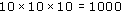Powers notation can be used to write 1000 = 103.

Two tens are multiplied together to give one hundred (10 × 10 = 100) so the superscript after the 10 must be 2, i.e. 102.

When expressing 100 and 1000 in powers of ten, there are no great savings on writing zeros, but what about one million (1000 000)? One million is the product of multiplying together six tens: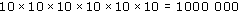so it is written as 106. Now you can begin to see the benefit of the powers of ten notation.

One thousand is often written not just as 103 but as 1 × 103. Spoken aloud, this is 'one times ten to the power three' or just 'one times ten to the three'. Likewise, one million is either 1 × 106 or simply 106. Now two alternative explanations can be given that may help you to grasp powers of ten. The power of ten shows how many times 1 has been multiplied by 10. Taking 1 × 103 as an example, 1000 is seen to be 1 × 10 × 10 × 10. In a second view, the power of ten shows how many places the decimal point has to move to the right to give the actual number. If 1 is written as 1.0 to remind you where the decimal point is, one move to the right would turn 1.0 into 10.0, a second move would give 100.0 and a third move would give 1000.0, i.e. one thousand.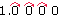You do not have to recall both of these ways of understanding powers of ten; just use the one that suits you best, or develop your own way of fixing the idea in your armoury of mathematical techniques.

Using the powers of ten notation, the total amount of water on Earth - 1460 000 000 cubic kilometres - could be written as 1.46 × 109 km3. A significant saving on zeros! The complete number would be spoken as 'one point four six times ten to the power nine' or just 'one point four six times ten to the nine'. The '9' in 109 tells you how many times 1.46 has been multiplied by 10 to give the final number of 1460 000 000. It is nine times, i.e. the number comprises: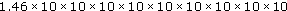To see clearly that this expression is still one thousand four hundred and sixty million, it helps to begin with 1.46 and to then multiply each time by ten to get the number you want:

1.46

1.46 × 10      = 14.6 = 1.46 × 101

1.46 × 10 × 10   = 146 = 1.46 × 102

1.46 × 10 × 10 × 10 = 1460 = 1.46 × 103

If you carry on doing this, you will end up with: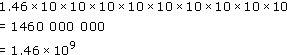Alternatively, you can think of each increase by one in the power of ten as moving the decimal point one place to the right. That is, if you multiply 1.46 by 10 the decimal point moves one place to the right, giving 14.6.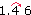Likewise, to multiply 1.46 by one thousand, the decimal point moves three places to the right, giving 1460.0. In the powers of ten notation, this is written as 1.46 × 103.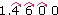The convention called scientific notation is used when writing a number with a power of ten. Scientific notation requires the number accompanying the power of ten to be less than 10 but equal to or greater than 1. Take the example of one million. It could be correctly expressed as 1 × 106, 10 × 105, 100 × 104, 1000 × 103, and so on, or even as 0.1 × 107 but only the first of these obeys the convention of scientific notation and this is the one that should be used. As a second example, it is mathematically correct to write 85 000 as 85 × 103 or 0.85 × 105 but correct scientific notation would demand 8.5 × 104.

Scientific notation requires the number accompanying the power of ten to be less than 10 but equal to or greater than 1.

## Question 2

Express the following numbers in scientific notation.

(a) 100 000 000

(b) 35 000

(c) 95 × 105

(d) 0.51 × 103

(a) 100 000 000 = 1 × 108

(b) 35 000 = 3.5 × 104

(c) 95 × 105 = 9.5 × 106

(d) 0.51 × 103 = 5.1 × 102

## Question 3

Write out in full the numbers corresponding to:

(a) 7.3 × 104

(b) 4.44 × 105

(c) 6.05 × 103